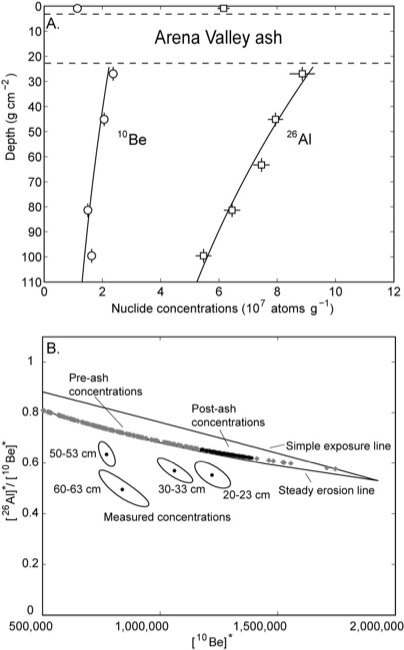Fig. 4. Data for the Arena Valley ash siteView Original Image at Full Size

Figure 4. Measured 10Be (circles) and 26Al (squares) concentrations (atoms g-1quartz) at the Arena Valley ash site. The small vertical and horizontal lines indicate the range of depth of the sediment sampled and the error in the nuclide measurement, respectively. In some cases, these values are smaller than the markers used to indicate each datum. The white box shows the approximate location of the Arena Valley ash in the soil column. The solid lines show the predicted nuclide concentrations for the best-fit model results where the erosion rate after the emplacement of the ash was ~0.2 m Ma-1.

4.B) A Klein-Nishiizumi-two-isotope diagram, which plots the 26Al/10Be ratio against the 10Be concentration. Nuclide concentrations for each sample have been divided by the production rate of the nuclides at each sample depth. For each sample that we have concentrations of both 10Be and 26Al, the measurements are shown as black circles with ellipses around them representing the 68% confidence interval. The general interpretation of this diagram is that cosmogenic nuclide concentrations that reflect only surface exposure plot on the upper line, concentrations that reflect erosion plot on the lower line, and concentrations that reflect a more complicated exposure history, such as burial or inheritance, plot below these lines. A more detailed explanation of this diagram can be found in Lal (1991). The grey boxes and black circles show the results of using an exposure model to consider the nuclide concentrations as the sum of their pre- and post-ash components, respectively. Both pre-and post-ash components plot directly on the steady erosion line, indicating that the measured nuclide concentrations can be explained by erosion alone and do not indicate that the sample site has been buried.

Image 18431 is a 1340 by 832 pixel JPEG